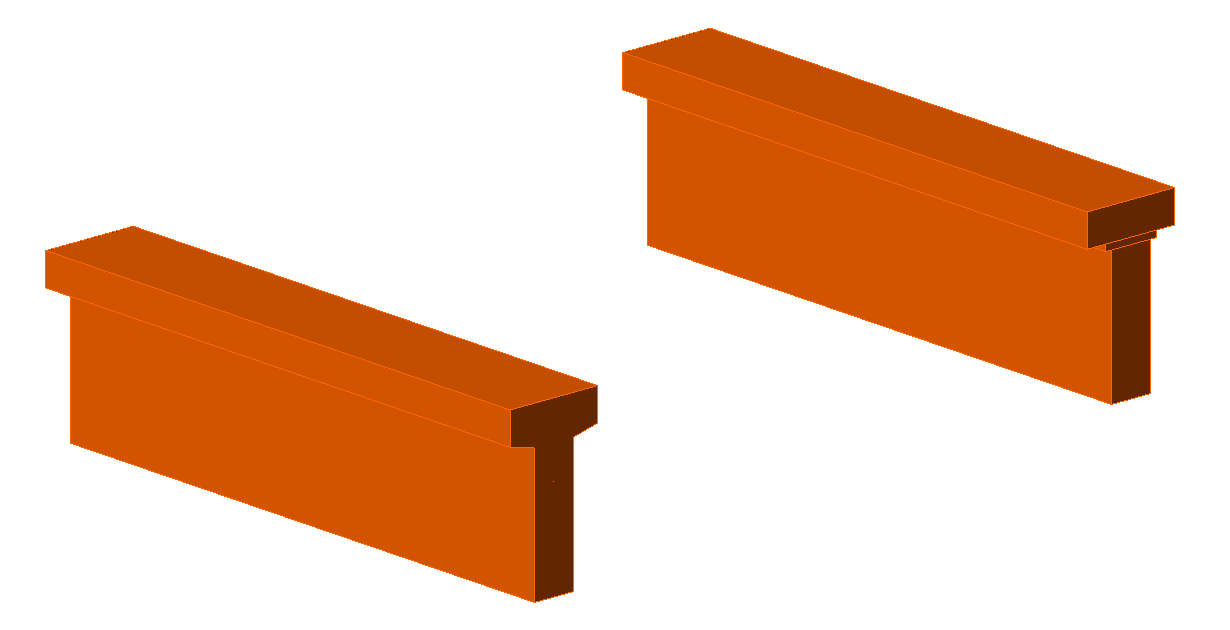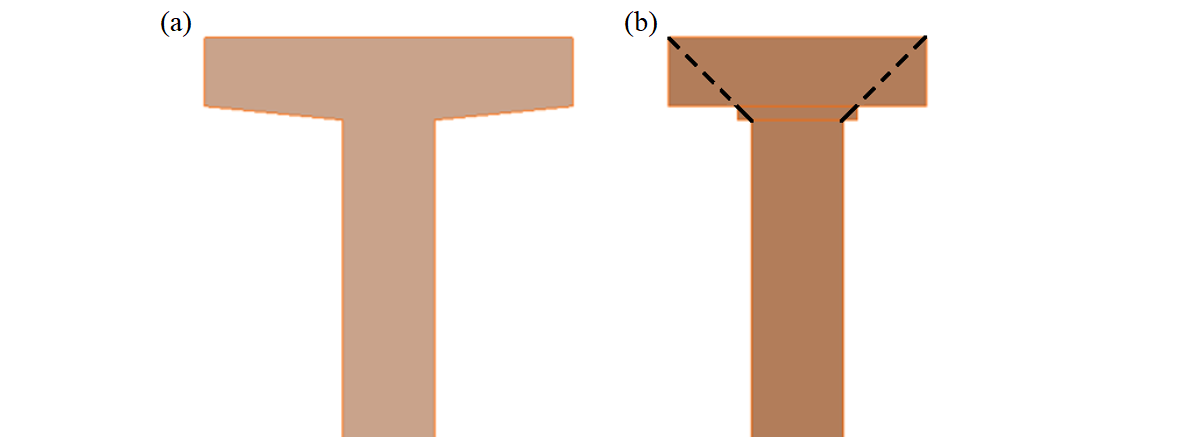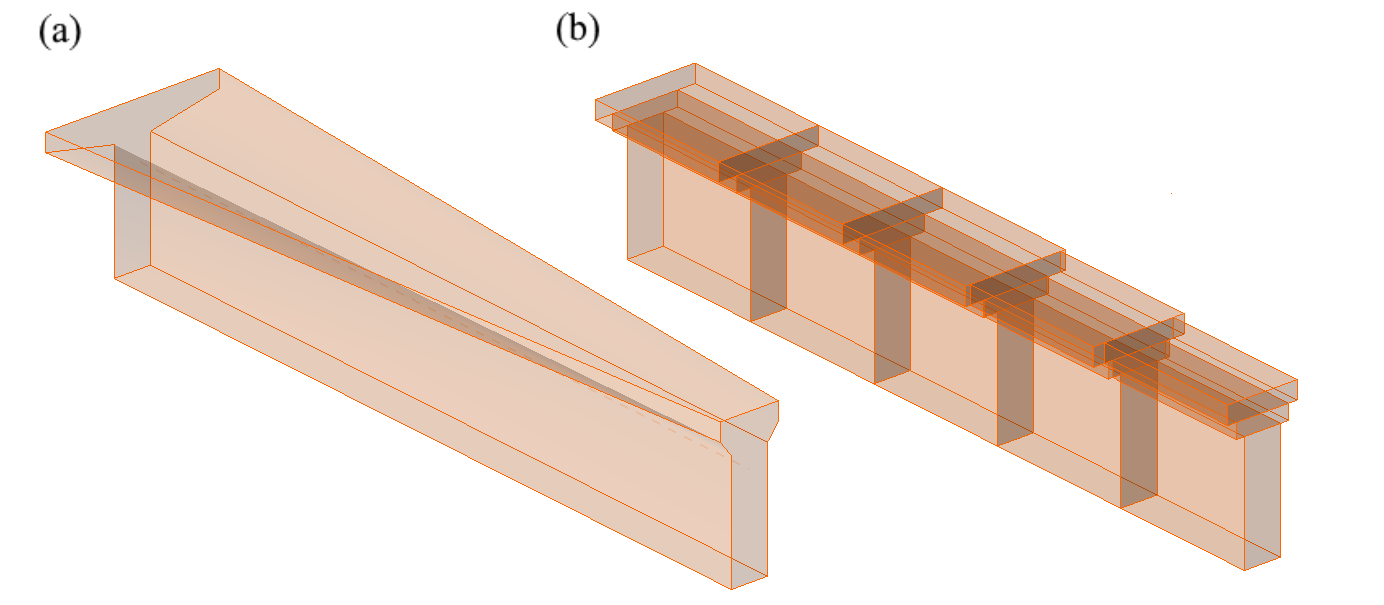### Choose language# Geometric modification of cross-sections

$$In the case of geometries which include haunches, the width of the wall elements used to model the haunch is reduced in comparison to the original width so that it is equal to double its height plus the thickness of the adjacent wall. This is based on the assumption that a compression stress field would expand from the wall at a 45° angle (see Fig. 13), so the aforementioned reduced width would be the maximal width capable of transferring loads.Note that the method of determining effective width flange implemented in CSFM is different from the one stated in 5.3.2.1 EN 1992-1-1 (2015). Besides geometry, Eurocode based effective width flange is explicitly affected by the span lengths and boundary conditions of a structure.$\textsf{\textit{\footnotesize{Fig. 13\qquad Width reduction of a cross-section: (a) user input; (b) FE model – automatically determined reduced flange width.}}}$In the case of haunches lying in the horizontal plane (Fig. 14), each haunch is divided into five sections along its length. Each of these sections is then modeled as a wall with a constant thickness, which is equal to the real thickness in the middle of the respective section.$\textsf{\textit{\footnotesize{Fig. 14\qquad Horizontal haunch: (a) user input; (b) FE model – a haunch automatically divided into five sections.}}}$$$